Chapter 1: Maya and European Dates The Maya Calendar

1. Long count dates

The Mayas used three different calendrical systems (and some variations within the systems). The three systems are known as the tzolkin (the sacred calendar), the haab (the civil calendar) and the long count system. The tzolkin is a cycle of 260 days and the haab is a cycle of 365 days (these cycles are explained in Sections 2 and 3 of this chapter). The tzolkin cycle and the haab cycle were combined to produce a cycle of 18,980 days, known as the calendar round. 18,980 days is a little less than 52 solar years. Thus the Mayas could not simply use a tzolkin/haab date to identify a day within a period of several hundred years because there would be several days within this period with the same tzolkin/haab date.

The Mayas overcame this problem by using a third dating system which enabled them to identify a day uniquely within a period of 1,872,000 days (approximately 5,125.36 solar years). To do this they used a vigesimal (i.e. based on 20) place-value number system, analogous to our decimal place-value number system.

The Mayas used a pure vigesimal system for counting objects but modified this when counting days. In a pure vigesimal system each place in a number is occupied by a number from 0 to 19, and that number is understood as being multiplied by a power of 20. Thus in such a system:

```    2.3.4    = 2*20*20 + 3*20 + 4*1 = 864
11.12.13 = 11*20*20 + 12*20 + 13*1 = 4653 and
1.3.5.7  = 1*20*20*20 + 3*20*20 + 5*20 + 7*1 = 9307```

When counting days, however, the Mayas used a system in which the first place (as usual) had a value of 1, the second place had a value of 20, but the third place had a value not of 400 (20*20) but of 360 (18*20). (This may have been due to the fact that 360 is close to the length of the year in days.) The value of higher places continued regularly with 7,200 (20*18*20), 144,000 (20*20*18*20), etc. In such a system:

```     1.3.5.7       = 1*20*18*20 + 3*18*20 + 5*20 + 7*1 = 8,387
and 11.12.13.14.15 = 11*20*20*18*20 + 12*20*18*20 + 13*18*20 + 14*20 + 15*1
= 11*144,000 + 12*7,200 + 13*360 + 14*20 + 15
= 1,675,375.```

A Maya long count date is a modified vigesimal number (as described above) composed of five places, e.g. 9.11.16.0.0, and interpreted as a count of days from some base date. There are many long count dates inscribed in the stellae and written in the codices. Calculation of the decimal equivalent of a long count yields a number of days. This is regarded as a number of days counted forward from a certain day in the past. It is the number of days since the day 0.0.0.0.0. The obvious question is: What day was used as the base date? This question has two aspects: (1) What day was used by the Mayas as the base date? (2)  What day was that in terms of the Western calendar? We shall return to these questions below.

Just as we have names (such as week) for certain periods of time, the Mayas had names for periods consisting of 20 days, 360 days, 7,200 days, etc., in accord with their modified vigesimal system of counting days. A day is known as a kin. Twenty kins make a uinal, 18 uinals a tun, 20 tuns a katun and 20 katuns a baktun. Thus we have:

```            1 kin    =  1 day
1 uinal  = 20 kins   = 20 days
1 tun    = 18 uinals = 360 days
1 katun  = 20 tuns   = 7,200 days
1 baktun = 20 katuns = 144,000 days```

The numbers at the five places in the long count are thus counts of baktuns, etc., as follows:

`baktuns . katuns . tuns . uninals . kin`

Thus, for example, 9.15.9.0.1 denotes a count of 9 baktuns, 15 katuns, 9 tuns, no uinals and 1 kin, or in other words, 9*144,000 + 15*7,200 + 9*360 + 0*20 + 1*1 days, or 1,407,241 days. It is a count of days from the Maya base date of 0.0.0.0.0.

Most of the long count dates which occur in the stone inscriptions have a baktun count of 9. The period 9.0.0.0.0 through 10.0.0.0.0, the period of the Classic Maya, is now thought by scholars to coincide with the period (approximately) 436 A.D. through 829 A.D. There are, however, some strange anomalies. Morley  deciphers two long count dates (found at Palenque) as 1.18.5.4.0 and 1.18.5.3.6 (14 days apart) which are some 2,794 solar years prior to 9.0.0.0.0. Since there is no evidence that the Mayas existed before about 500 B.C., what could these early long count dates possibly be referring to?

We would expect that the next higher unit after the baktun would consist of 20 baktuns, and it appears there was such a unit, called a pictun. However, no long count date occurs with a baktun count of more than 12, except that 13.0.0.0.0 occurs (see Morley , pp.203-4, for an example). A widely-accepted school of thought holds that in the Maya long count system 13.0.0.0.0 marks the beginning of a new cycle, and so is equivalent to 0.0.0.0.0. In this view, 13 baktuns make up a great cycle or, Maya era, of 13*144,000 = 1,872,000 days (approximately 5125.37 solar years).

Here is the date sequence for the entire 12.19.7 tun (this was produced using the Mayan Calendrics software).

2. Tzolkin dates

The tzolkin, sometimes known as the sacred calendar, is a cycle of 260 days. Each tzolkin day is denoted by a combination of a number from 1 through 13 and a name from the following set of twenty (in the order: Imix, Ik, Akbal, Kan ....):

```Imix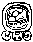Cimi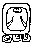ChuenCibIkManik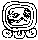EbCaban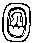AkbalLamatBen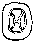EdznabKan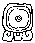MulucIx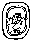Cauac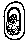Chicchan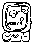Oc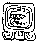Men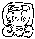Ahau```

The days cycle through the numbers and through the names independently. The sequence of tzolkin days thus runs:

```            1 Imix
2 Ik
3 Akbal
4 Kan
. . .
13 Ben
1 Ix        (here we repeat the cycle of numbers)
2 Men
3 Cib
4 Caban
5 Edznab
6 Cauac
7 Ahau
8 Imix      (here we repeat the cycle of names)
9 Ik
10 Akbal
. . .```

There are 260 elements in this sequence. That is because 260 is the least common multiple of 13 and 20. Thus the cycle of (13) tzolkin day numbers combined with (20) tzolkin day names repeats each 260 days.

In order to explain this 260-day calendrical cycle some have speculated that the Mayas chose this number of days because their allegedly advanced astronomical knowledge revealed to them that a period of 260 days fits well with certain astronomical periods, such as the eclipse-year. A more prosaic explanation is that there were originally two branches of Maya society, one of which used a 13-day cycle of numbered days and the other a 20-day cycle of named days. (There is a set of thirteen Maya gods, which may be the origin of the 13 numbered days, similar to our week.) Then at some point in early Maya history the two groups merged, combining the two calendars so that neither group would lose their method of day-reckoning, resulting in the 260-day cycle as described above.

3. Haab dates

The Mayas also maintained a so-called "civil" calendar, called the "haab". This was similar to our calendar in that it consisted of months, and within months, of days numbered consecutively. However, unlike our calendar, the haab cycle is made up of eighteen months of twenty days each, plus five days at the end of the year. The eighteen names for the months (in the order: Pop, Uo, Zip ...) are:

```PopXulZacPax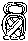UoYaxkin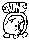Ceh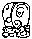Kayab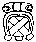Zip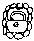Mol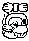Mac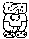Cumku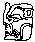Zodz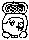Chen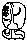Kankin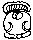Zec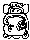Yax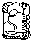Muan```The five extra days formed the "month" of Uayeb, meaning "nameless". The five "nameless" days were considered unlucky. One did not get married in Uayeb. The haab cycle thus consisted of 18*20 + 5 = 365 days, the integral number of days closest to the mean solar year of 365.2422 mean solar days.

The sequence of days from the first day of the year to the last thus runs as follows:

```    0   Pop
1   Pop
...
19  Pop
0   Zip
1   Zip
...
19  Zip
0   Zodz
...
19  Cumku
0   Uayeb
...
4   Uayeb```

For most of Maya history the first day of Pop was denoted by 0 Pop and the last by 19 Pop. However, on the eve of the Spanish conquest the first day of Pop began to be numbered 1, and the last day 20 (except for Uayeb), so that the year began with 1 Pop and ended with 5 Uayeb.

There is some uncertainty as to whether (what has usually been taken to be) the first day of each haab month (e.g., 0 Zip) is really the last (i.e., the 20th, or the 5th) day of the preceding month (Pop in this case), or in other words, whether the last day of each month was actually written as "the day before the beginning of (the next) month", where the glyph translated as "the seating of" was used with the meaning of "the day before the beginning of the next month, namely ...". 0 Zip can be interpreted either as the first day of Zip or as the last day of Pop, but unfortunately the classic Maya are no longer here to tell us how they understood this date.

4. The Maya calendar round

The tzolkin and the haab are each cycles of days; the former is a cycle of 260 days and the latter is a cycle of 365 days. When specifying a day the Maya usually used both the tzolkin date and the haab date, as in 4 Ahau 3 Kankin. For the Mayas these two cycles ran together and concurrently, as shown by the following sequence of days:

``` Tzolkin date    Haab date
10 Ben          11 Kayab
11 Ix           12 Kayab
12 Men          13 Kayab
13 Cib          14 Kayab
1 Caban         15 Kayab
2 Edznab        16 Kayab
3 Cauac         17 Kayab
4 Ahau          18 Kayab
5 Imix          19 Kayab
6 Ik            0 Cumku
7 Akbal         1 Cumku
8 Kan           2 Cumku
. . .
12 Imix         19 Cumku
13 Ik           0 Uayeb
1 Akbal         1 Uayeb
2 Kan           2 Uayeb
3 Chicchan      3 Uayeb
4 Cimi          4 Uayeb
5 Manik         0 Pop
6 Lamat         1 Pop
7 Muluc         2 Pop
...```

Since 260 = 4*5*13 and 365 = 5*73, the earliest that a tzolkin/haab date combination can repeat is after 4*5*13*73 = 18,980 days, or just short of 52 solar years. This cycle of 18,980 days is called the Maya calendar round.

Maya long count dates are often given in association with the corresponding tzolkin/haab date, as in:

```         8.11.7.13.5    3 Chicchan 8 Kankin
10.1.19.15.17   12 Caban 0 Yax
10.3.8.14.4     6 Kan 0 Pop
10.6.2.0.9      9 Muluc 7 Yax
10.6.10.12.16   3 Cib 9 Uo```

A particular tzolkin/haab date recurs every 18,980 days, whereas a long count date (assuming that the long count starts over at 0.0.0.0.0 on reaching 13.0.0.0.0) recurs every 1,872,000 days (once in 5,125.37 years). The combination of a long count date and a tzolkin/haab date occurs only once every 136,656,000 days (approximately 374,152 years or 73 Maya eras).

5. Julian dates

The Julian calendar, introduced by Julius Caesar in 46 B.C., is the basis of our modern calendar. It consists of a system of twelve months, January, February, etc. (although New Year's Day has not always been January 1st). If the number of the year is divisible by 4 then February has 29 days, otherwise it has 28. A date in the Julian calendar is termed a Julian date.

The Romans identified their years as a number of years supposed to have elapsed since the founding of Rome (which we now date as having occurred in 753 B.C.) Following the merger (under Constantine) of the Christian Church and the Roman Imperium years came to be numbered with reference to the year of the birth of Christ (now regarded as actually having occurred in 4 B.C.) In this system the year immediately before the year 1 A.D. is the year 1 B.C.

Astronomers use a system, which is also used in Mayan Calendrics, in which the year prior to the year 1 is the year 0. Thus 1 B.C. is the year 0, 2 B.C. is the year -1, 3 B.C. is the year -2, and so on. More generally the year n B.C. in common usage is said by astronomers to be the year -(n-1). (See more on this in section 7.)

According to Aveni , p.127, "the serial numbering of the years as we know them did not actually begin until the sixth century ..." Thus dates prior to 600 are always uncertain. The Emperor Augustus also tinkered with the lengths of the months during his reign, introducing a further element of uncertainty, and it is also possible that the Council of Nicea (325 A.D.) readjusted the calendar by a couple of days.

6. Gregorian dates

The average length of a year in the Julian calendar is 365.25 days, differing from the value of the mean solar year by about .0078 days. This resulted in a slow shift of the Julian calendrical year with respect to the solar year (i.e. to the solstices and equinoxes). By the 16th Century the Julian calendar was seriously out of synch with the seasons and Pope Gregory XIII introduced the Gregorian Calendar. This involved three changes:

(a) The day following October 4, 1582, was declared to be October 15, 1582, thereby excising ten days from the calendar.

(b) A year was declared to be a leap year if (i) it was divisible by 4 but not by 100 or (ii) it was divisible by 400.

(c) New rules for determining the date of Easter were introduced.

The Gregorian Calendar is now commonly used throughout the West and is the de facto international common calendar. There have been numerous suggestions for replacing it with a more "rational" calendar, but old habits die hard and any change would be expensive.

7. Julian day numbers

Astronomers use a system of dating days known as the Julian day number system, in which a day is identified as that day which is a certain number of days before or after the day -4712-01-01 (January 1st, 4713 B.C.) in the Julian calendar. Thus, for example, the day whose Julian day number is 584,283 is September 6, -3113 in the Julian calendar, 584,283 days after January 1st, -4712 J. This day is also August 11th, -3113 in the Gregorian calendar. By 2001-01-01 G we will have reached the day whose Julian day number is 2,451,991, by which time nearly two-and-a-half million days will have elapsed since -4712-01-01 J.